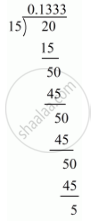# Express the Following Rational Number as Decimal: -2/15 - Mathematics

Express the following rational number as decimal:

-2/15

#### Solution

Given rational number is -2/15

Now we have to express this rational number into decimal form. So we will use long division methodTherefore 2/15=0.1333

rArr2/15=0.1bar3

Hence, -2/15=-0.1bar3

Is there an error in this question or solution?
Chapter 1: Number Systems - Exercise 1.2 [Page 13]

#### APPEARS IN

RD Sharma Mathematics for Class 9
Chapter 1 Number Systems
Exercise 1.2 | Q 2.3 | Page 13
Share